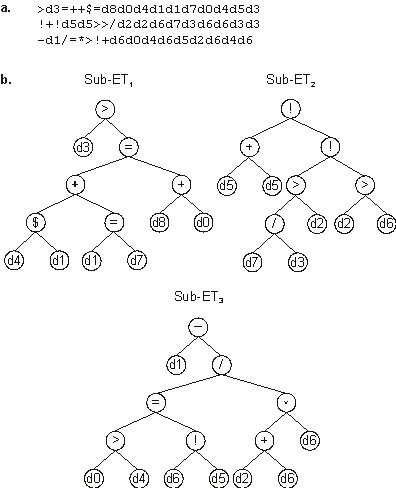Home
News
Author
Q&A
Tutorials
GEP Biblio
Contacts

Visit Gepsoft

Gene Expression Programming: Mathematical Modeling by an Artificial Intelligence

Diagnosis of breast cancer

In this diagnosis task the goal is to classify a tumor as either benign (0) or malignant (1) based on nine different cell analysis (input attributes or terminals).

The model presented here was obtained using the cancer1 dataset of PROBEN1 where the binary 1-of-m encoding in which each bit represents one of the m-possible output classes was replaced by a 1-bit encoding (“0” for benign and “1” for malignant). The first 350 samples were used for training and the last 174 were used for testing the performance of the model in real use. This means that absolutely no information from the testing set samples or the testing set performance are available during the adaptive process. Thus, the classification error on the testing set is a good measure to evaluate the generalization performance.

For this problem, F = {+, -, *, /, <, >, :, \$, =, !}, but each function was weighted twice (the last six symbols are comparison functions of two arguments which return the value of the first argument if true or the second if false, representing, respectively, less than, greater than, less than or equal to, equal to or greater than, equal to, and not equal to); the set of terminals consisted of the nine attributes used in this problem and were represented by T = {d0, ..., d8} which correspond, respectively, to clump thickness, uniformity of cell size, uniformity of cell shape, marginal adhesion, single epithelial cell size, bare nuclei, bland chromatin, normal nucleoli, and mitoses.

In classification problems where the output is often binary, it is important to set criteria to convert real-valued numbers into zero or one. This is the 0/1 rounding threshold Rt that converts the output of a chromosome into one if the output is equal to or greater than Rt, or into zero otherwise. For this problem we are going to use Rt = 0.

The fitness function in classification problems is usually very simple. The fitness fi of an individual program corresponds to the number of hits and is evaluated by the equation:

 if n > Cp, then fi = n; else fi = 0 (4.28)

where n is the number of fitness cases correctly evaluated, and Cp is the number of fitness cases of the class with more members (predominant class).

For this problem, chromosomes composed of three genes with an h = 8 and sub-ETs linked by addition were used. The program below was discovered using a small population of 50 individuals:

 >d3=++\$=d8d0d4d1d1d7d0d4d5d3  !+!d5d5>>/d2d2d6d7d3d6d6d3d3 -d1/=*>!+d6d0d4d6d5d2d6d4d6 (4.29a)

It has a fitness of 340 evaluated against the training set of 350 fitness cases and a fitness of 173 evaluated against the testing set of 174 examples. This corresponds to a testing set classification error (the percent of incorrectly classified examples) of 0.575% and a classification accuracy of 97.143%.

Note that for the expression of this chromosome to be complete the 0/1 rounding threshold Rt = 0 must be taken into account. With the software APS we can automatically convert the model (4.29) above into a fully expressed C++ function:

 double APSCfunction(double d[]) { double dblTemp = 0; dblTemp += (d>(((d>=d?d:d)+ (d==d?d:d))==(d+d)?((d>=d?d:d)+ (d==d?d:d)):(d+d))?d:(((d>=d?d:d)+ (d==d?d:d))==(d+d)?((d>=d?d:d)+ (d==d?d:d)):(d+d))); dblTemp += ((d+d)!=(((d/d)>d?(d/d):d)!= (d>d?d:d)?((d/d)>d? (d/d):d):(d>d?d:d))? (d+d):(((d/d)>d?(d/d):d)!= (d>d?d:d)?((d/d)>d? (d/d):d):(d>d?d:d))); dblTemp += (d-(((d>d?d:d)==(d!=d? d:d)?(d>d?d:d):(d!=d? d:d))/((d+d)*d))); return (dblTemp >= 0 ? 1 : 0); } (4.29b)

In this form, the model seems really complicated, but its parsing shows that its sub-ETs are really simple (Figure 4.13). Note that all the attributes seem to be relevant to an accurate diagnosis of breast cancer. Indeed, one of the advantages of the models evolved by GEP is that they allow knowledge extraction because they are not only accessible but also easy to interpret.Figure 4.13. Model evolved by GEP to diagnose breast cancer. a) The three-genic chromosome encoding sub-ETs linked by addition. b) The sub-ETs codified by each gene. Note that the expression of this chromosome is only complete after including the rounding threshold which in this case is equal to zero.

Home | Contents | Previous | Next